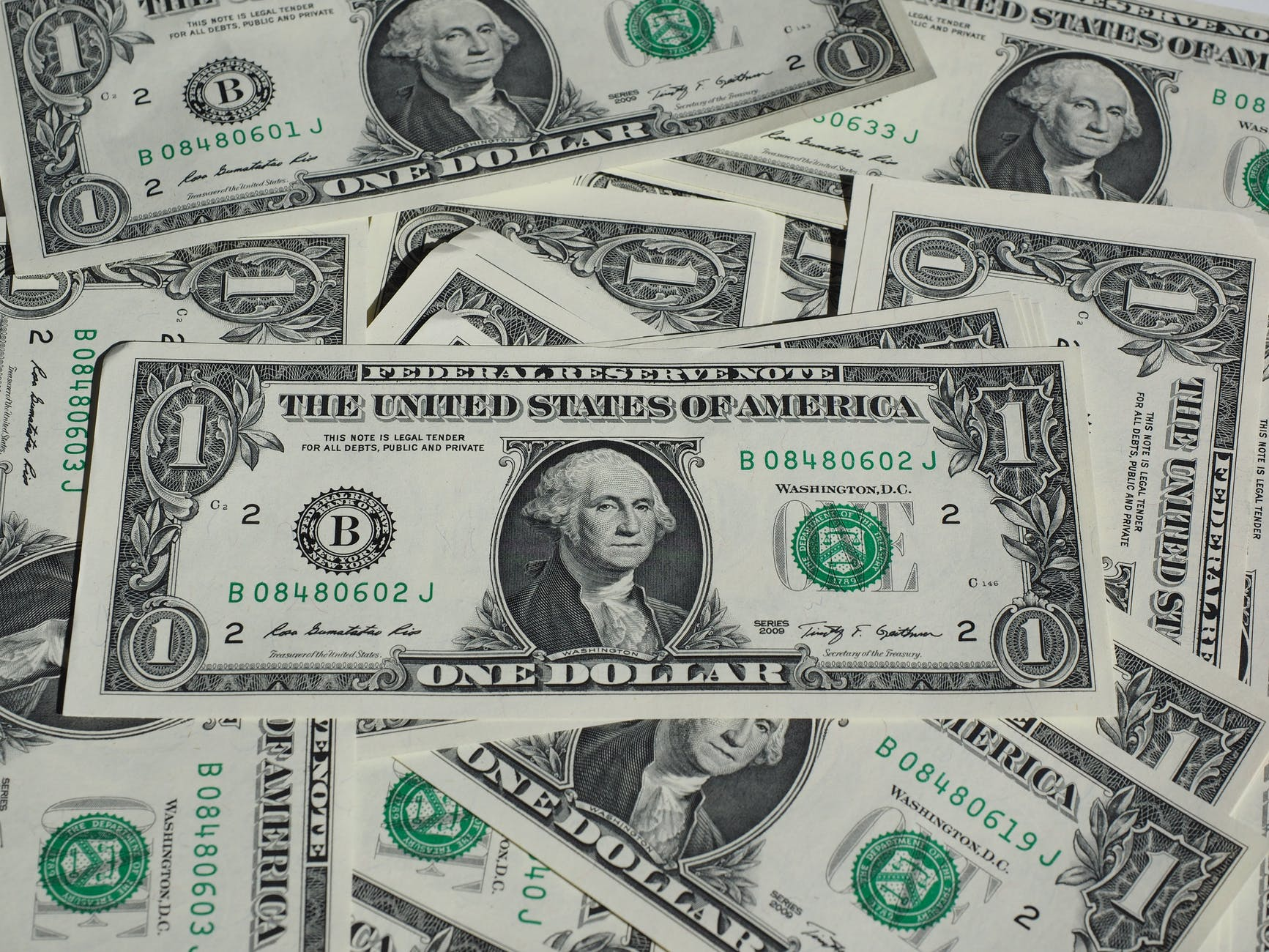# Total CostBasics
The formula provided for total cost by the Mathematics Formula Sheet is straightforward, but it can be rearranged to ask questions about price per unit and number of units, too.

A question involving total cost is often a plug-in (where you end up plugging in numbers for words).

The word per is a hint that a cost-related question could be coming.

Formulas (Mathematics Formula Sheet)
Total Cost = [Number of Units] × [Cost Per Unit]

Formulas (NOT on Mathematics Formula Sheet)
Cost Per Unit = [Total Cost] ÷ [Number of Units]

Number of Units = [Total Cost] ÷ [Cost Per Unit]

Question

A bakery charges \$0.79 per doughnut.  What is the total cost of 12 doughnuts?

\$9.48

[Number of Units] = 12 doughnuts
[Cost Per Unit] = \$0.79 per doughnut
Total Cost = [Number of Units] × [Cost Per Unit]
Total Cost = 12 × 0.79 = 9.48

Input Display Commentblinker clears screen
12 × 0.79 12*0.79 Total Cost =
Number of Units × Cost Per Unit9.48 Answer
###### Total Cost

Question
A crew worked from 6 AM to 7 PM replacing a faulty roof at a cost of \$8,124.22.  How much was the crew paid per hour?

\$624.94 per hour

[Total Cost] = \$8124.22
[Number of Units] = 6 AM to 7 PM = 13 hours
Cost Per Unit = [Total Cost] ÷ [Number of Units]
Cost Per Unit = 8124.22 ÷ 13 = 624.94

Input Display Commentblinker clears screen
8124.22 ÷ 13 8124.22÷13 Cost Per Unit =
Total Cost ÷ Number of Units624.94 Answer
###### Total Cost: Cost Per Unit

Question
A concession stand sold \$375.00 worth of popcorn.  The popcorn cost \$1.25 per bag.  How many bags of popcorn did the concession stand sell?

300 bags

[Total Cost] = \$375.00
[Cost Per Unit] = \$1.25 per bag
Number of Units = [Total Cost] ÷ [Cost Per Unit]
Number of Units = 375.00 ÷ 1.25 = 300

Input Display Commentblinker clears screen
375.00 ÷ 11 375.00÷1.25 Number of Units =
Total Cost ÷ Cost Per Unit300 Answer
###### Total Cost: Number of Units

Practice – Questions

1.  A chili supper is held at a cost of \$5.00 per person.  What is the total cost of 150 people?

2.  A fast food restaurant charges 99 cents per hamburger.  What is the total cost of 25 hamburgers?

3.  Bob rented a snowmobile from 7 AM to 6 PM at a cost of \$244.75.  What was the rental cost per hour?

4.  Betty rented a car for 5 days at a cost \$199.75.  What was the rental cost per day?

5.  A celebrity earned \$5050.00 signing autographs.  The celebrity charged \$50.00 per autograph.  How many autographs did the celebrity sign?